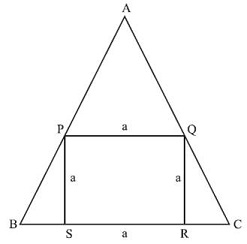# CAT Quantitative Aptitude Questions | Geometry Questions for CAT - Triangles

###### CAT Questions | CAT Geometry Questions | Equilateral Triangle and Square

The question is from CAT Geometry - Triangles. It discusses about an equilateral triangle with a square inscribed inside it. CAT Geometry questions are heavily tested in CAT exam. Make sure you master Geometry problems. This question asks about ratio of area of the square to that of the equilateral triangle.

Question 6: There is an equilateral triangle with a square inscribed inside it. One of the sides of the square lies on a side of the equilateral △. What is the ratio of the area of the square to that of the equilateral triangle?

1. 12 : 12 + 7√3
2. 24 : 24 + 7√3
3. 18 : 12 + 15√3
4. 6 : 6 + 5√3

## Best CAT Coaching in Chennai

#### CAT Coaching in Chennai - CAT 2022Limited Seats Available - Register Now!

##### Method of solving this CAT Question from Triangles: Mathematicians love symmetry, question setters are nearly besotted with it. So, learn about symmetric figures well.APQ is an equilateral △. As PQ is parallel to BC.
Let side of the square be ‘a’
AP = a = AQ
△QRC has angles 30 – 60 – 90.

$$frac{QR}{QC}\\$ = $\frac{√3}{2}\\$ QC = QR * $\frac{2}{√3}\\$ AC = AQ + QC = a + $\frac{2a}{√3}\\$ Area of equilateral △ = $\frac{√3}{4}\\$ AC2 = $\frac{√3}{4}\\$$a + $$frac{2a}{√3}\\$)2 = $\frac{√3}{4}\\$$$$frac{√3a + 2a}{√3}\\$)2 = $\frac{√3}{4}\\$ *$$$frac{1}{3}\\$) a2$4 + 3 + 4√3)
= $$frac{7 +4 √3}{12}\\$ * √3a2 = $\frac{12 + 7√3}{12}\\$ * a2 Ratio of area of square to that of equilateral △ is = a2 : $\frac{12 + 7√3}{12}\\$ * a2 = 12 : 12 + 7√3 The question is "What is the ratio of the area of the square to that of the equilateral triangle?" ##### Hence, the answer is 12 : 12 + 7√3. Choice A is the correct answer. ###### Prepare for CAT 2023 with 2IIM's Daily Preparation Schedule ###### Know all about CAT Exam Syllabus and what to expect in CAT ###### Best CAT Online Coaching Try upto 40 hours for free Learn from the best! ###### Already have an Account? ###### CAT Coaching in ChennaiCAT 2023 Classroom Batches Starting Now! @Gopalapuram ###### Best CAT Coaching in Chennai Introductory offer of 5000/- Attend a Demo Class ###### Best Indore IPM & Rohtak IPM CoachingSignup and sample 9 full classes for free. Register now! ## CAT Preparation Online | CAT Geometry questions Videos On YouTube #### Other useful sources for Geometry Question | Geometry Triangles Circles Quadrilaterals Sample Questions ##### Where is 2IIM located? 2IIM Online CAT Coaching A Fermat Education Initiative, 58/16, Indira Gandhi Street, Kaveri Rangan Nagar, Saligramam, Chennai 600 093 ##### How to reach 2IIM? Phone:$91) 44 4505 8484
Mobile: (91) 99626 48484
WhatsApp: WhatsApp Now
Email: prep@2iim.com